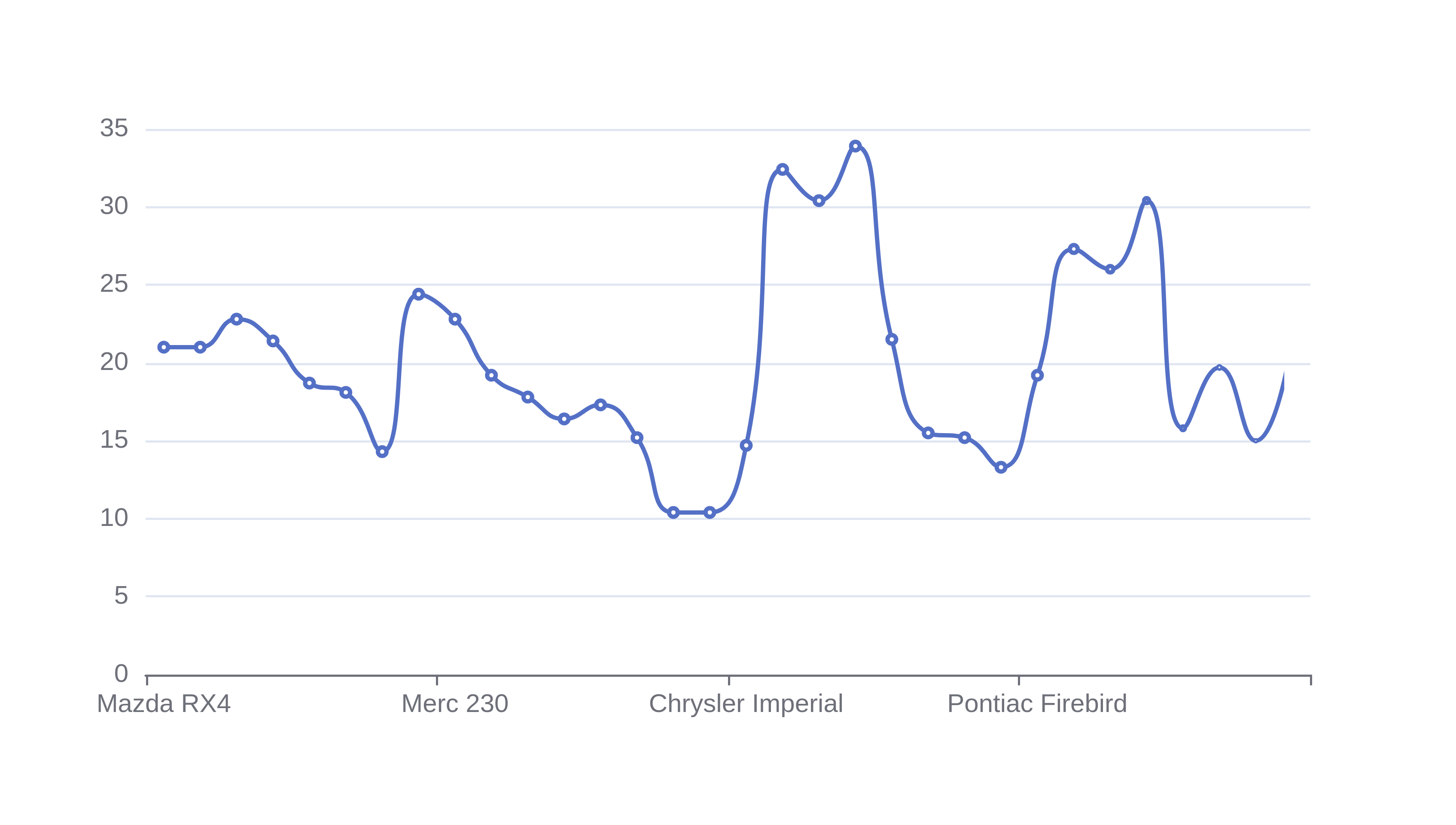# Graph Maker

Or input your data as csv

Sharing helps us build more free tools

## Types of Graphs and Charts

This online graph maker can make the following data visualizations from raw data.

Data can be imported from csv, tsv, json or parquet data files. Click the feedback button if you would like more ways to import data.

### Histogram

The histogram maker can create histograms from numerical data.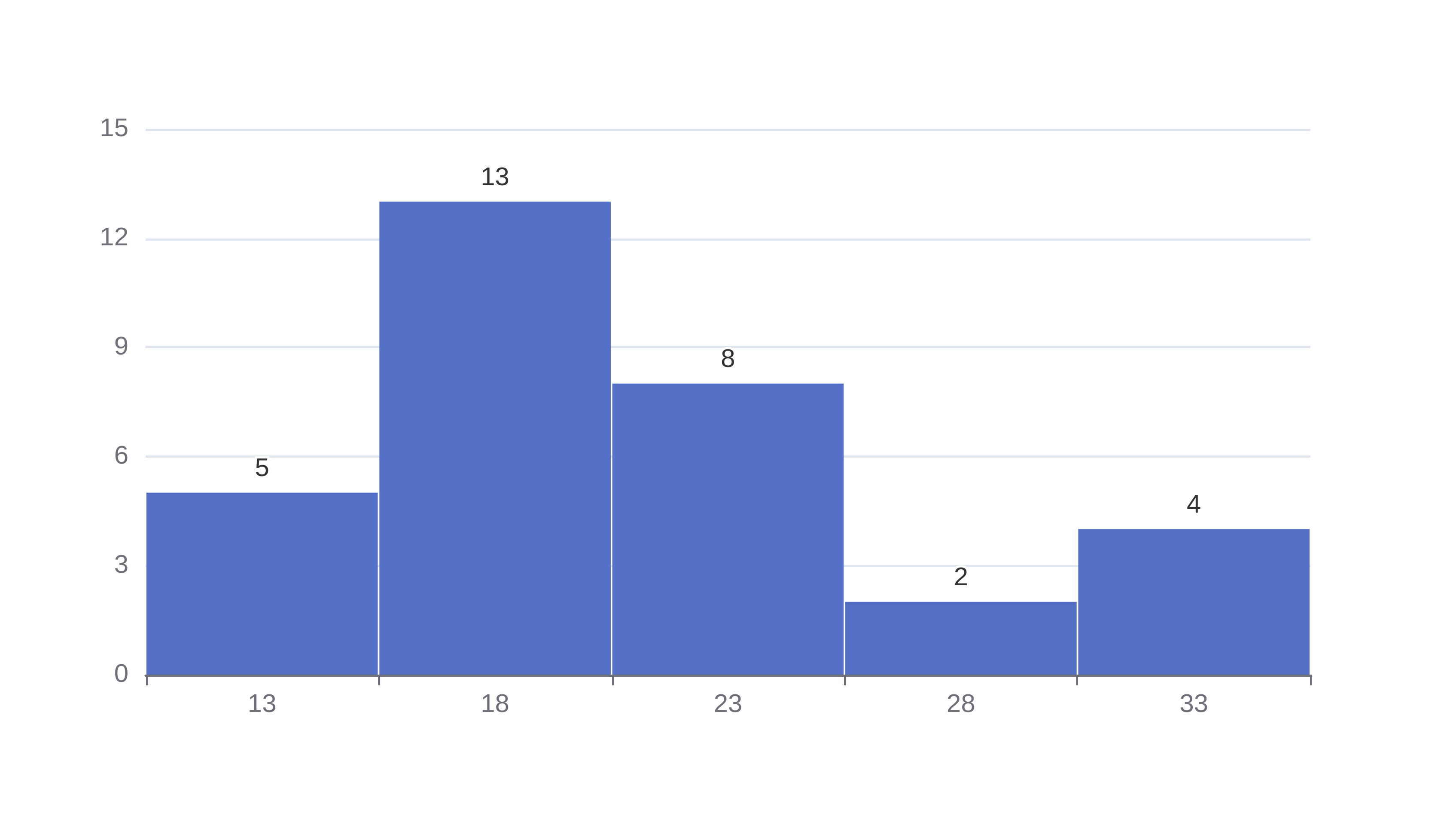### Bar Graphs

The bar graph maker can build bar charts from numerical and qualitative data.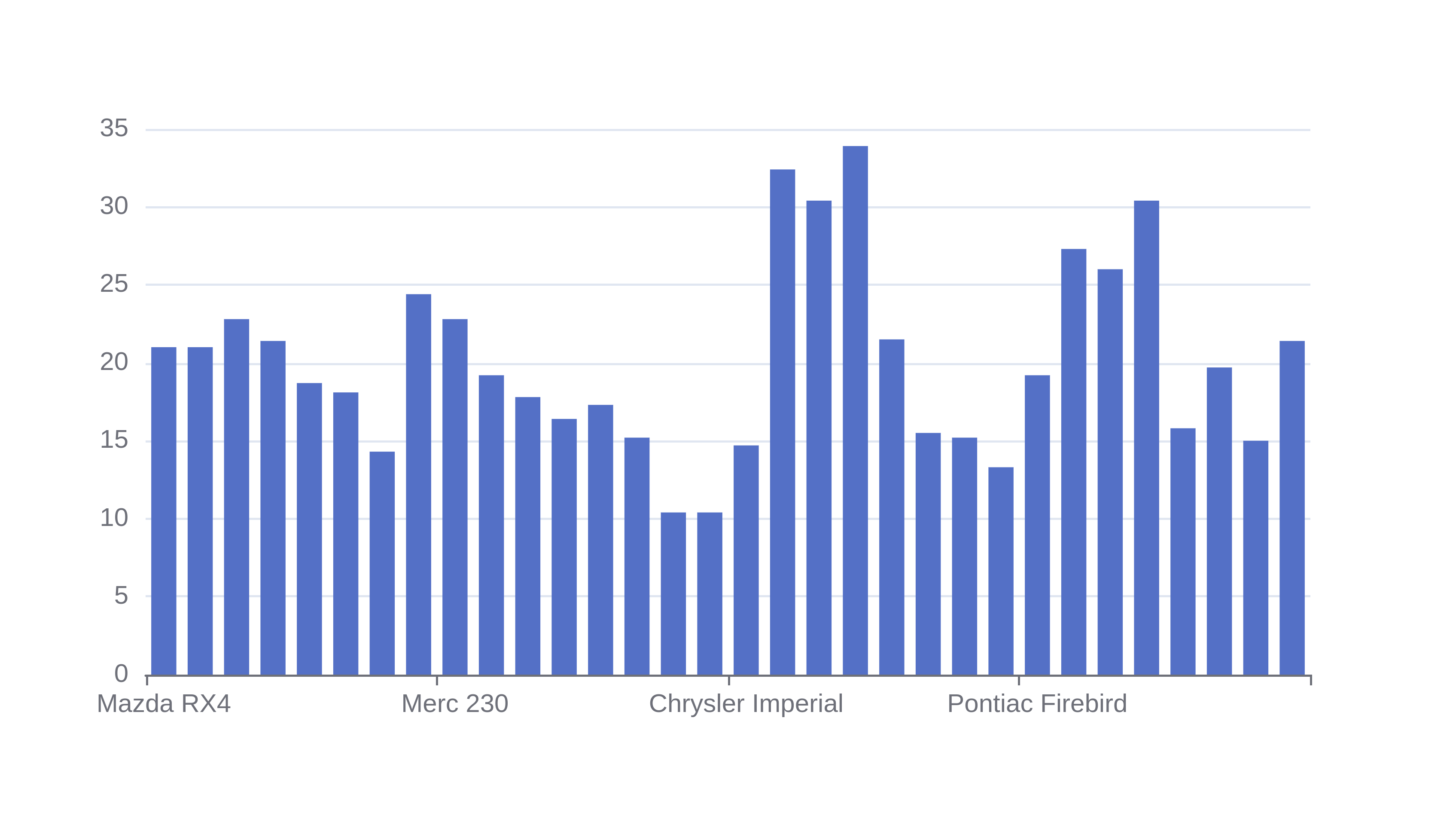### Area Graphs

The area graph maker creates area charts from numerical and categorical data.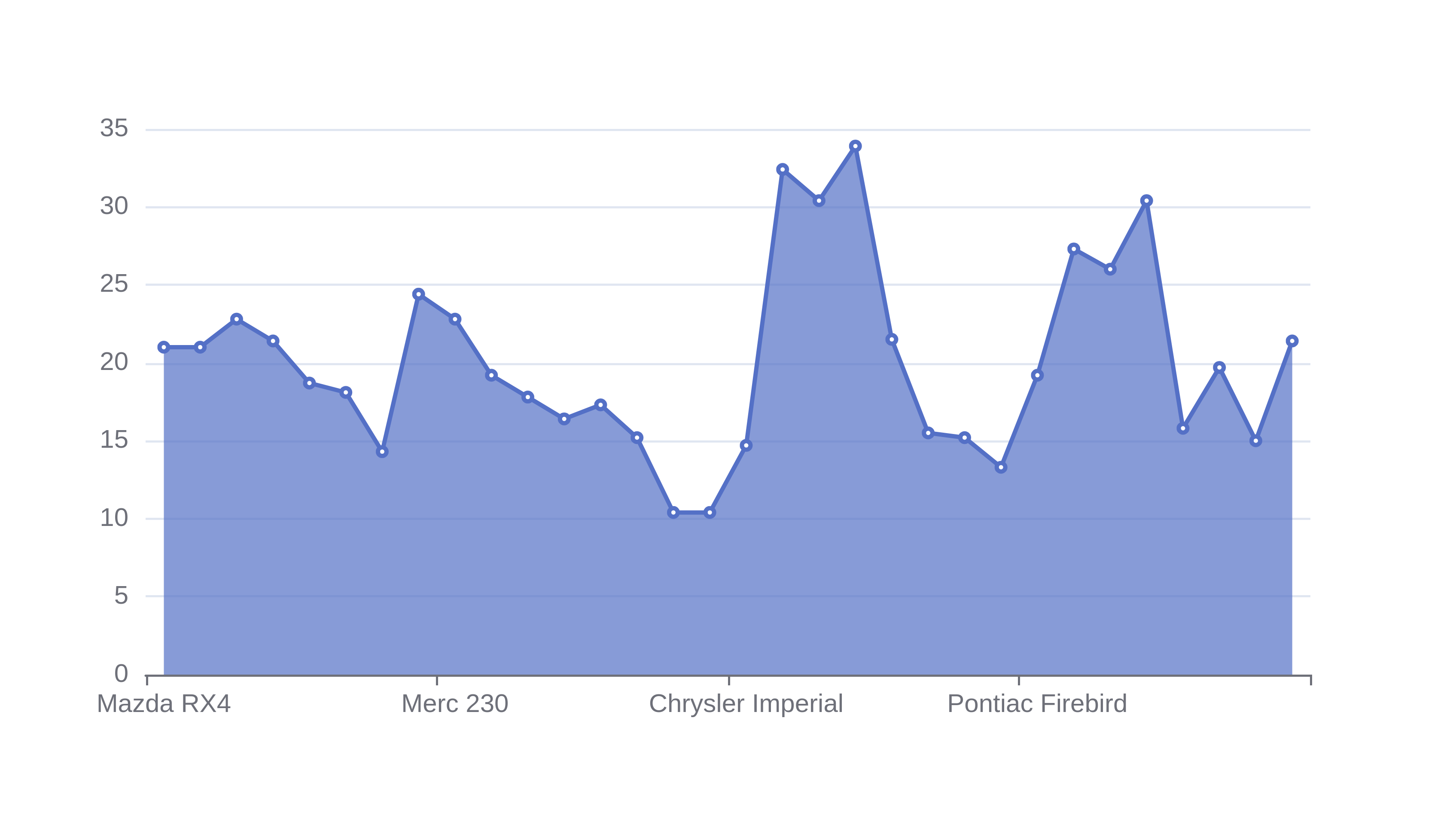### Scatter Plot

The scatter plot maker creates scatter plots from numerical and categorical raw data.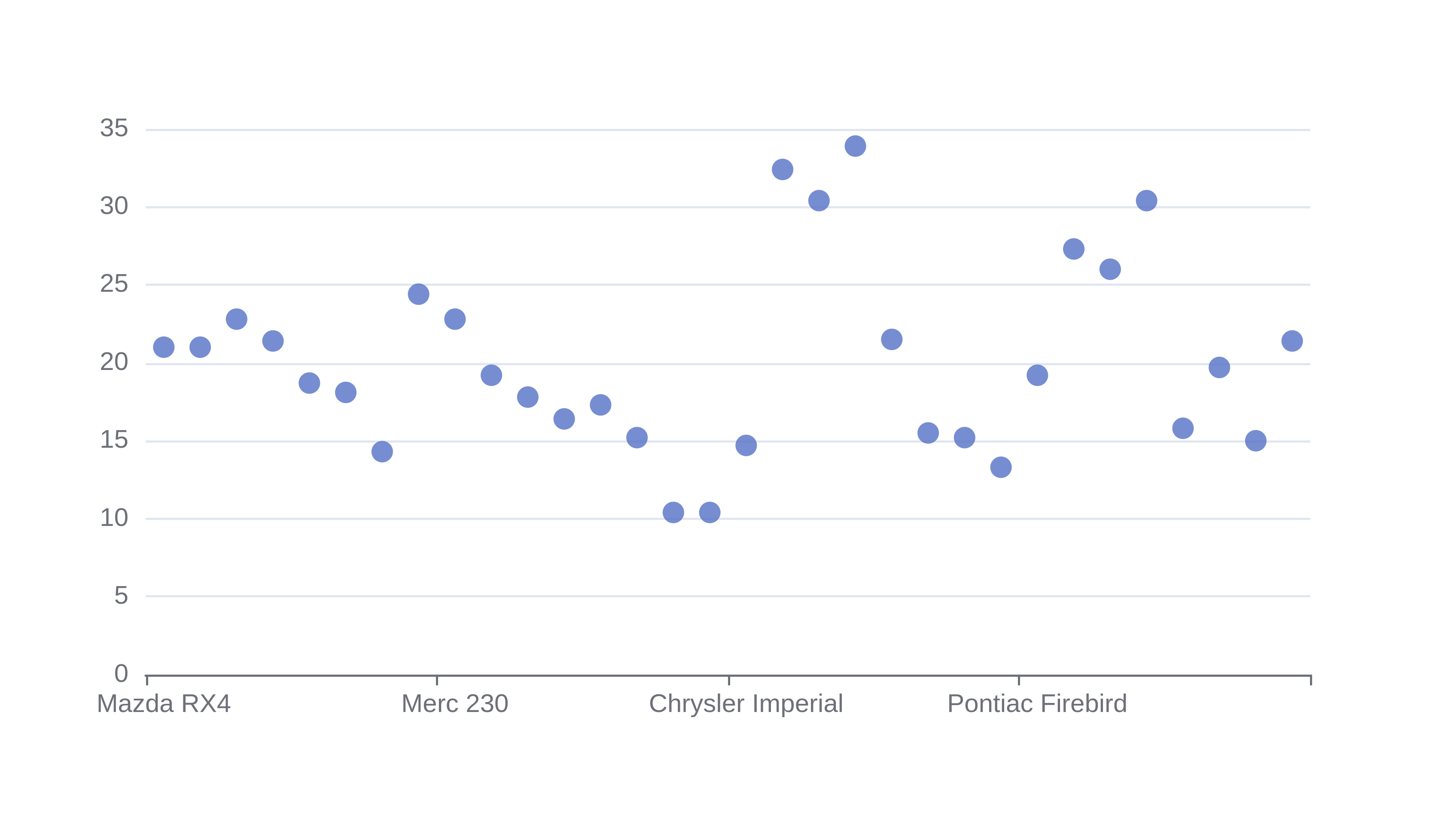### Pie Charts

The Pie chart maker creates pie charts from numerical and categorical data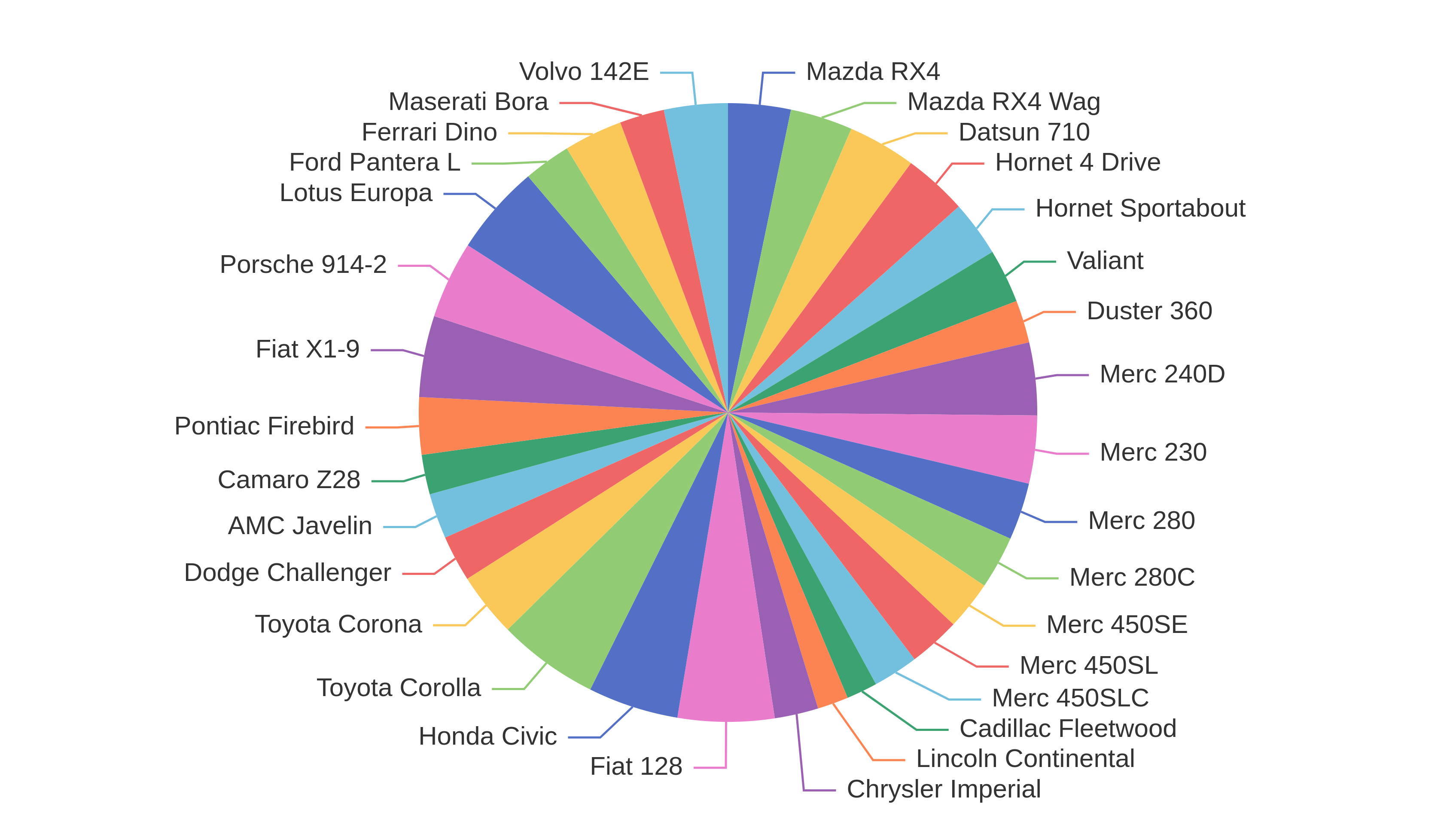### Box and Whisker Plot

The box and whisker plot maker creates graphs from numerical data.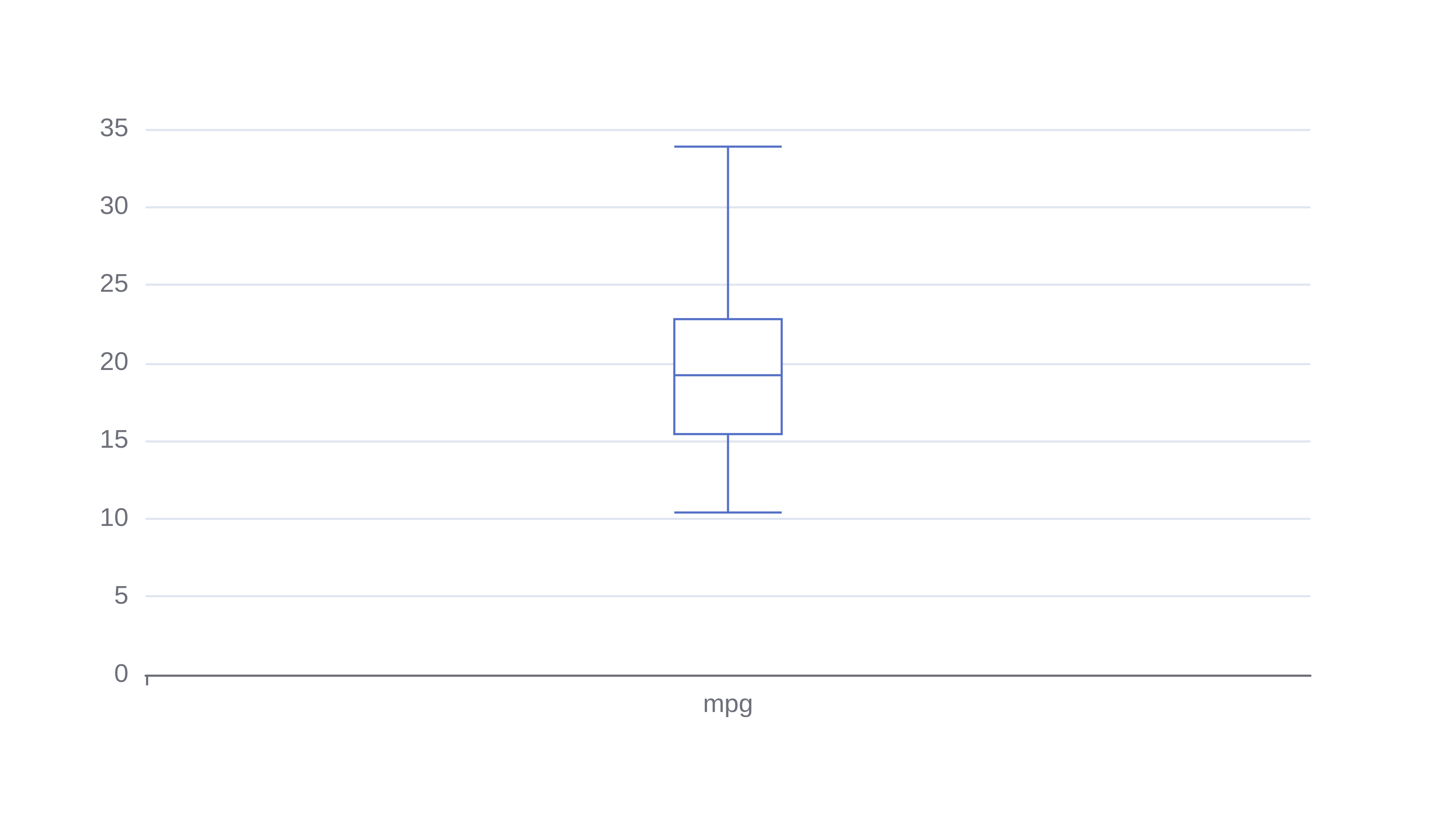### Area Spline Graph

The area spline graph maker creates graphs from numerical and categorical data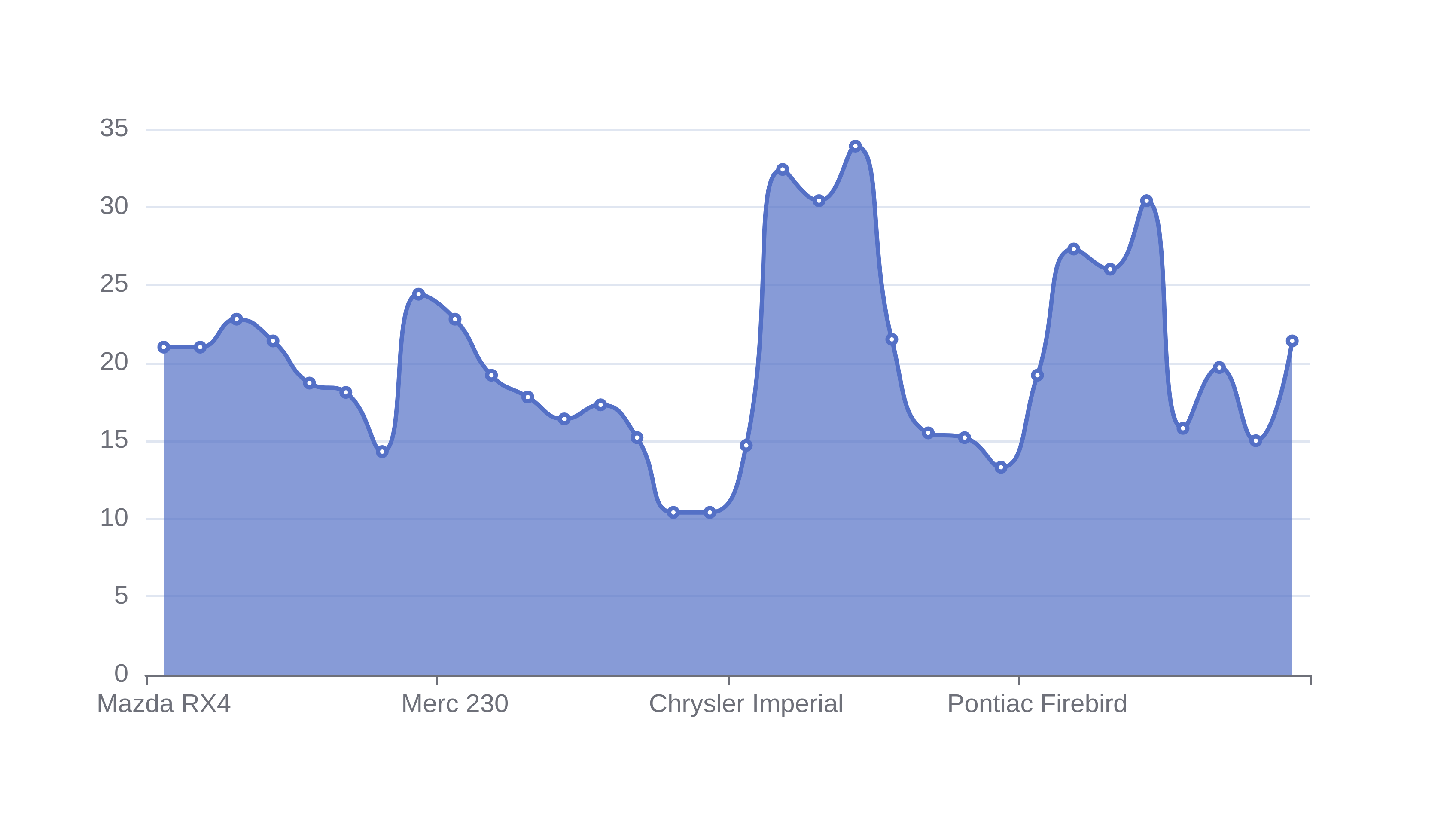### Line Graphs

The line graph maker creates graphs from numerical and categorical data.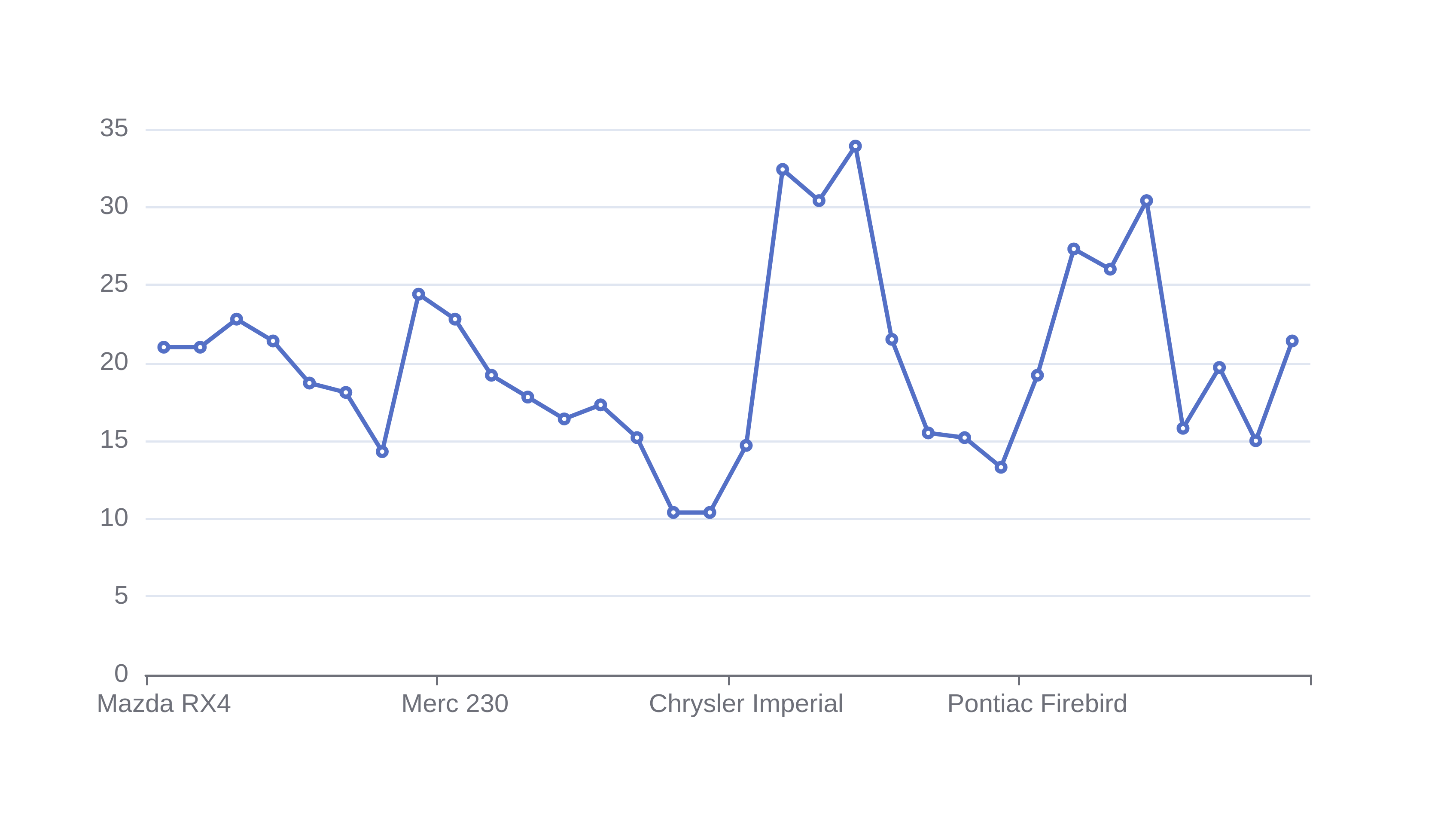### Spline Graphs

The spline graph maker builds spline graphs from numerical and categorical data